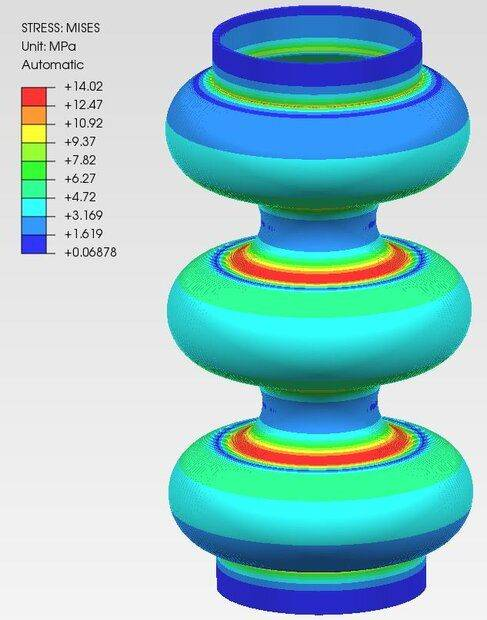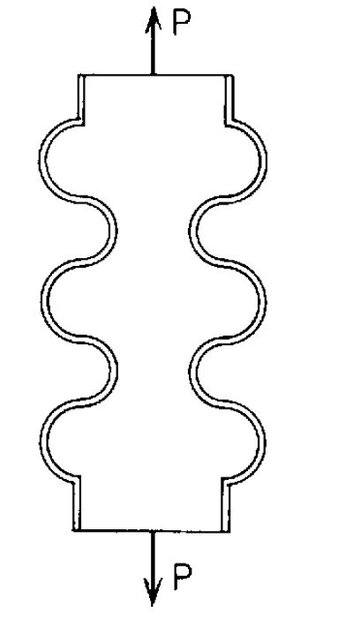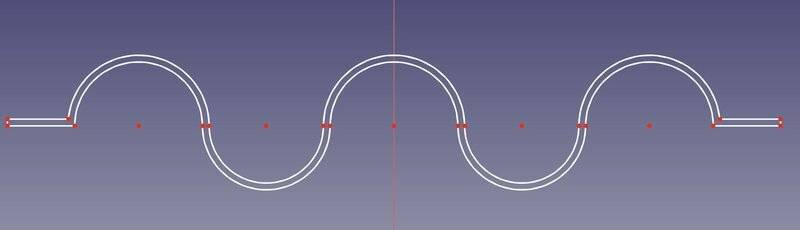# Corrugated tube (bellow) under axial load

• FEAnalyst
In summary, the conversation discusses verifying the results of a finite element analysis of a corrugated tube subjected to axial load. The analytical calculations used are based on Roark's Formulas for Stress and Strain. The values used in the calculations are provided and the results are compared to the results from the finite element analysis. There is also a discussion about the assumptions made in the analytical solution and how they may affect the geometry of the tube. The conversation ends with the clarification that this is a benchmark problem for educational purposes and will not be manufactured.f

#### FEAnalyst

TL;DR Summary
What can be wrong with my calculations of a corrugated tube subjected to axial load since there's no agreement with FEA?
Hi,

I'm trying to verify the results of a finite element analysis of a corrugated tube (bellow) subjected to axial load. Here are my analytical calculations based on Roark's Formulas for Stress and Strain (originally the formulas are from the article "On the Theory of Thin Elastic Toroidal Shells" by R.A. Clark): $$u=\frac{0.577Pbn \sqrt{1-\nu^{2}}}{E t^{2}}$$ $$\sigma_{m,max}=\frac{1.63P}{2 \pi at} \left[ \frac{ab}{t^{2} \sqrt{1-\nu^{2}}} \right]^{1/3}$$ $$\sigma_{h,max}=\frac{0.925P}{2 \pi at} \left[ \frac{ab(1- \nu^{2})}{t^{2}} \right]^{1/3}$$ where: ##P## - axial load, ##a## - distance from the axis of the tube to the center of the semicircular corrugation, ##b## - outer radius of the semicircular corrugation, ##t## - wall thickness, ##n## - number of corrugations, ##E## - Young's modulus, ##\nu## - Poisson's ratio, ##u## - stretch, ##\sigma_{m,max}## - maximum meridional bending stress, ##\sigma_{h,max}## - maximum circumferential (hoop) membrane stress. In my case the values are: $$P=2000 \ N, \ a=80 \ mm, \ b=42 \ mm, \ t=4 \ mm, \ n=5, \ E=210 \ GPa, \ \nu=0.3$$ and I got the following results from the formulas above: $$u=0.0688 \ mm$$ $$\sigma_{1,max}=9.79 \ MPa$$ $$\sigma_{2,max}=5.29 \ MPa$$ I also calculated von Mises stress (for direct comparison with FEA) using this formula (it should be correct but I'm not 100% sure): $$\sigma_{vM}=\sqrt{\frac{1}{2} \left( (\sigma_{r}- \sigma_{h})^{2}+(\sigma_{h}- \sigma_{m})^{2}+(\sigma_{m}- \sigma_{r})^{2} \right) }=8.48 \ MPa$$ This was calculated assuming radial stress ##\sigma_{r}=0##. The calculations themselves are correct because I performed them using CAS (Computer Algebra System) software.

Here's what I got from axisymmetric FEA: $$u=0.07986 \ mm$$ $$\sigma_{vM}=14.02 \ MPa$$ And that's the stress distribution:Do you know what can be wrong here ? I double-checked all the calculations. The FEA model is very simple and there should be no problem with boundary conditions for example.

Do you know why Roark calls the corrugation "semicircular"?
Do you know what calculation method is used by axisymmetric FEA?

Do you have a specific application for this?
I ask because the wall thickness should vary along the longitude of the shape, depending on the method of manufacture and stretching of the material.

Thank you for the reply. According to Roark's the tube should look like this:And here's the sketch used to create the geometry (2D surface) for the analysis:I just drew 5 tangent circles with aligned centers and equal radii. Then I trimmed them using the line passing through the centers to get semicircles and added straight lines at the ends. Finally, I used the offset function to apply constant wall thickness to the section and converted the sketch to a surface. This should agree with assumptions in the book but I also wondered if the geometry is fully correct. Do you think that it should be modified somehow considering the assumptions of the analytical solution ?

This is just a benchmark problem for education purposes, the tube won't be manufactured.

In axisymmetric FEA it's assumed that the geometry, loading and boundary conditions are all constant around the axis. The analysis is actually performed on a 2D model (surface) but then in postprocessing it can be revolved to visualize the results in 3D (that's what I did in the first post).

•Lnewqban